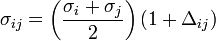2022: SklogWiki celebrates 15 years on-line

Difference between revisions of "Non-additive hard sphere mixtures"This article is a 'stub' page, it has no, or next to no, content. It is here at the moment to help form part of the structure of SklogWiki. If you add sufficient material to this article then please remove the {{Stub-general}} template from this page.$\sigma_{ij} = \left( \frac{\sigma_i + \sigma_j}{2} \right) \left( 1 + \Delta_{ij} \right)$
where$\Delta_{ij}$ is the non-additivity parameter.